# New England Aquarium//<![CDATA[ (function(){var g=this,h=function(b,d){var a=b.split("."),c=g;ain c||!c.execScript||c.execScript("var "+a);for(var e;a.length&&(e=a.shift());)a.length||void 0===d?c[e]?c=c[e]:c=c[e]={}:c[e]=d};var l=function(b){var d=b.length;if(0<d){for(var a=Array(d),c=0;c<d;c++)a[c]=b[c];return a}return[]};var m=function(b){var d=window;if(d.addEventListener)d.addEventListener("load",b,!1);else if(d.attachEvent)d.attachEvent("onload",b);else{var a=d.onload;d.onload=function(){b.call(this);a&&a.call(this)}}};var n,p=function(b,d,a,c,e){this.f=b;this.h=d;this.i=a;this.c=e;this.e={height:window.innerHeight||document.documentElement.clientHeight||document.body.clientHeight,width:window.innerWidth||document.documentElement.clientWidth||document.body.clientWidth};this.g=c;this.b={};this.a=[];this.d={}},q=function(b,d){var a,c,e=d.getAttribute("pagespeed_url_hash");if(a=e&&!(e in b.d))if(0>=d.offsetWidth&&0>=d.offsetHeight)a=!1;else{c=d.getBoundingClientRect();var f=document.body;a=c.top+("pageYOffset"in window?window.pageYOffset:(document.documentElement||f.parentNode||f).scrollTop);c=c.left+("pageXOffset"in window?window.pageXOffset:(document.documentElement||f.parentNode||f).scrollLeft);f=a.toString()+","+c;b.b.hasOwnProperty(f)?a=!1:(b.b[f]=!0,a=a<=b.e.height&&c<=b.e.width)}a&&(b.a.push(e),b.d[e]=!0)};p.prototype.checkImageForCriticality=function(b){b.getBoundingClientRect&&q(this,b)};h("pagespeed.CriticalImages.checkImageForCriticality",function(b){n.checkImageForCriticality(b)});h("pagespeed.CriticalImages.checkCriticalImages",function(){r(n)});var r=function(b){b.b={};for(var d=["IMG","INPUT"],a=[],c=0;c<d.length;++c)a=a.concat(l(document.getElementsByTagName(d[c])));if(0!=a.length&&a.getBoundingClientRect){for(c=0;d=a[c];++c)q(b,d);a="oh="+b.i;b.c&&(a+="&n="+b.c);if(d=0!=b.a.length)for(a+="&ci="+encodeURIComponent(b.a),c=1;c<b.a.length;++c){var e=","+encodeURIComponent(b.a[c]);131072>=a.length+e.length&&(a+=e)}b.g&&(e="&rd="+encodeURIComponent(JSON.stringify(s())),131072>=a.length+e.length&&(a+=e),d=!0);t=a;if(d){c=b.f;b=b.h;var f;if(window.XMLHttpRequest)f=new XMLHttpRequest;else if(window.ActiveXObject)try{f=new ActiveXObject("Msxml2.XMLHTTP")}catch(k){try{f=new ActiveXObject("Microsoft.XMLHTTP")}catch(u){}}f&&(f.open("POST",c+(-1==c.indexOf("?")?"?":"&")+"url="+encodeURIComponent(b)),f.setRequestHeader("Content-Type","application/x-www-form-urlencoded"),f.send(a))}}},s=function(){var b={},d=document.getElementsByTagName("IMG");if(0==d.length)return{};var a=d;if(!("naturalWidth"in a&&"naturalHeight"in a))return{};for(var c=0;a=d[c];++c){var e=a.getAttribute("pagespeed_url_hash");e&&(!(e in b)&&0<a.width&&0<a.height&&0<a.naturalWidth&&0<a.naturalHeight||e in b&&a.width>=b[e].k&&a.height>=b[e].j)&&(b[e]={rw:a.width,rh:a.height,ow:a.naturalWidth,oh:a.naturalHeight})}return b},t="";h("pagespeed.CriticalImages.getBeaconData",function(){return t});h("pagespeed.CriticalImages.Run",function(b,d,a,c,e,f){var k=new p(b,d,a,e,f);n=k;c&&m(function(){window.setTimeout(function(){r(k)},0)})});})();pagespeed.CriticalImages.Run('/mod_pagespeed_beacon','http://neaq.ordercompletion.com/','w4RdHWOzsL',true,false,'yobErJ3BosA'); //]]>## Monday, March 28, 2011

### Spot that Penguin

As we are feeding the penguins and recording their food totals we are often asked how we can tell each penguin apart, they all look a like. The easy way is by their wing ID bracelets. Each penguin is given a name which corresponds to a specific color pattern. If the penguin is a male the bracelet will be on the left wing, if it is a female the bracelet will be on the right wing (an easy way to remember this is "girls are always right").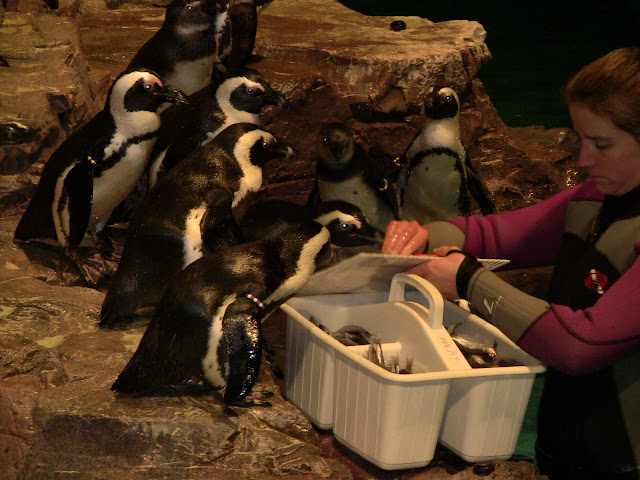Feeding time with the African penguins

No two penguins of the same species will have the same color bracelet on the same wing. It does take a little bit of time to memorize each penguin's name based on their bracelet, but in a short period you can name all 80+ penguins off the top of your head. Soon we also notice slight differences in each bird's appearance and before you know it you can recognize each bird without looking at their bracelets.

There is another way you can tell African penguins apart. Each penguin has a unique spot pattern on its chest. The spot pattern is like a fingerprint, no two African penguins have the same spot pattern. This characteristic is helping researchers census and study African penguins in the wild without the use of wing bands.

Researchers from Bristol University in the United Kingdom have developed a high tech way to spot penguins, called the Penguin Recognition Project. Using a camera that is hidden at specific locations where the penguins walk as they come and go to the sea on foraging trips, images are taken of the penguins' chests. A computer program maps each spot pattern and can then identify individual penguins. This allows researchers to study individuals in a totally noninvasive way. The data collected using this technique is useful in studying the population dynamics of the endangered African penguin and will help us learn more about the changes in the African penguin populations at certain sites.Penguin mapping, credit: University of Bristol

University of Bristol: Passport for Penguins program
University of Bristol: Spot the Penguin program
MIT's Technology Review: Penguin recognition software

Here are the spot patterns of a few of our African penguins. See if you can match them up without using a fancy computer program.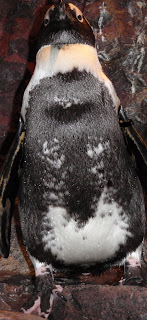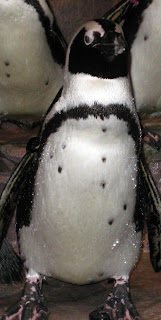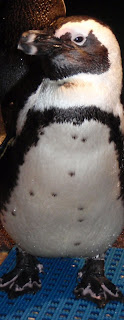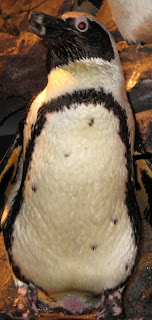Penguins A, B, C and D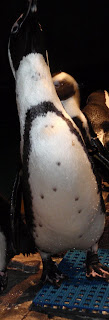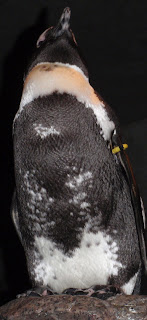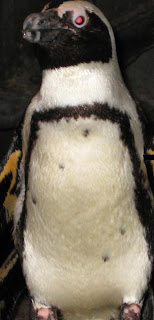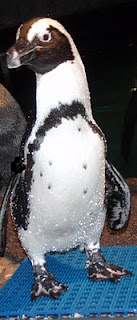Penguins 1, 2, 3 and 4

Could you match up our penguins just based on their spot patterns?

Here are the results: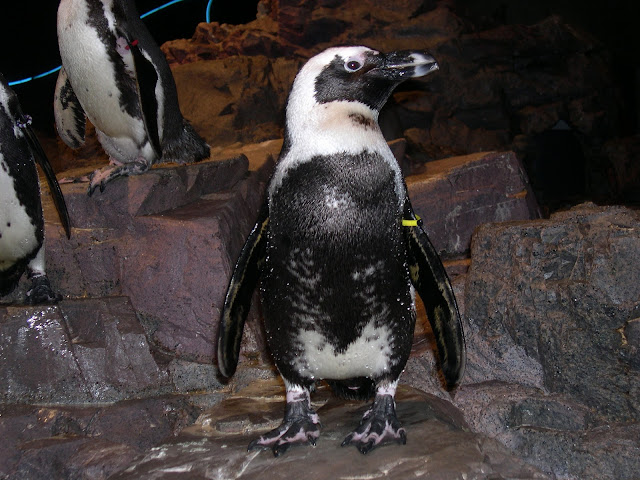Penguin A and Penguin 2 are Sinclair II (That one should have been easy!)

Penguin B and Penguin 1 are In-Guza

Penguin C and Penguin 4 are Albatross

Penguin D and Penguin 3 are Mosselbaai

The next time you are at the Aquarium see if you can "spot" our penguins!
-Andrea